# Grade - math word problems

#### Number of examples found: 5328

• FractionsThree-quarters of an unknown number are 4/5. What is 5/6 of this unknown number?
• Parking field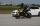There were nine cars in the parking field. The motorcycles were three times less. How many motorcycles are here and how many wheels are in the parking field?
• Unknown number 6Determine x if 1/6 of x is equal to 2/5 of the number 24.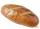5 of the same bread has the same weight as three bread and 4 kg of fruit. What weight has one bread?
• RectangleThe rectangle area is 182 dm2, its base is 14 dm. How long is the other side? Calculate its perimeter.
• Calculation of CNCalculate: ?
• Rhombus anglesIf one angle in the rhombus is 159°, what is it neighboring angle in rhombus?
• Find unknownFind unknown numerator: 4/8 + _/8 = 1
• One hectareHow many square meters are one hectare?
• Mixed2improperWrite the mixed number as an improper fraction. 166 2/3
• Divide moneyDivide 1200 USD at a ratio of 1:2:3:4:5:6:9:10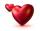Mom wants talk with dad 2.2 hours. But dad wants of 2 times more hours than mom ... How many hours wants dad talk with mom? ...
• Log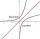Calculate value of expression log |3 +7i +5i2| .3 3/4 + 2 3/5 + 5 1/2 Show your solution.
• Two equationsSolve equations (use adding and subtracting of linear equations): -4x+11y=5 6x-11y=-5
• 75th percentile (quartille Q3)Find 75th percentile for 30,42,42,46,46,46,50,50,54
• Square roots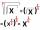What is equal to the product of the square roots of 295936?
• Cylinder diameter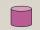The surface of the cylinder is 149 cm2. The cylinder height is 6 cm. What is the diameter of this cylinder?
• Area of trapezoidThe trapezoid bases are and 7 dm and 11 cm. His height is 4 cm. Calculate the area of trapezoid.
• Length subtractingExpress in mm: 5 3/10 cm - 2/5 mm

Do you have an interesting mathematical word problem that you can't solve it? Submit a math problem, and we can try to solve it.

We will send a solution to your e-mail address. Solved examples are also published here. Please enter the e-mail correctly and check whether you don't have a full mailbox.

Please do not submit problems from current active competitions such as Mathematical Olympiad, correspondence seminars etc...